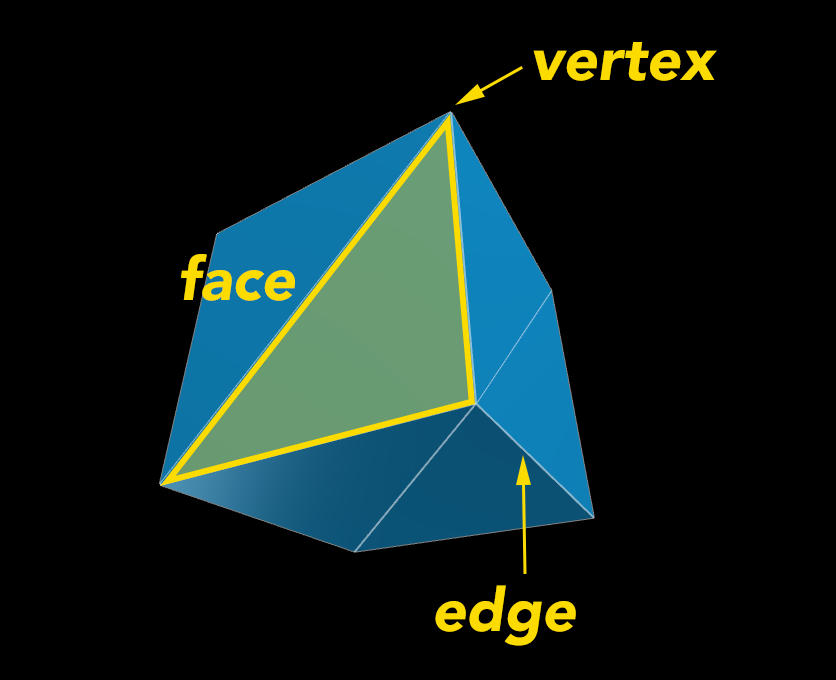This is part 2 in a series for beginners wanting to get in to using 3D WebGL. If you haven't checked out the other post be sure to have a look:

In the last post we looked at how to set up a Three.js scene with a camera and some lighting. The next step is to put some objects in the scene.

In order to get comfortable with adding 3D geometry to a WebGL scene it helps to understand a little 3D modelling terminology.

3D models (or meshes) are made up of vertices, edges and faces.

Vertices: the points of intersection between 3 edges.
Edges: an edge where two faces meet (think of the edges of a cube).
Faces: polygons (triangles) that make up the faces of the model.All 3D models (also named meshes) can be broken down in to these little triangle polygons. You could construct the model geometry yourself by specifying all of the vertice positions, but the great thing about Three.js is that it provides a bunch of methods for you to create primitive shapes.

Side Note: For my examples in this tutorial I'll be using a mesh normal material - I purposely won't address the material part because I want to keep the focus on geometry for this article, but we will cover materials in the future!

Let's see how you can add some geometry to a 3D scene

#### The code to create a cube

```  ```// create some geometry - this is how you create some square
// geometry using the BoxGeometry method
var geometry = new THREE.BoxGeometry( 20, 20, 20);
// create a material
var material = new THREE.MeshNormalMaterial();
// add the geometry to the mesh - and apply the material to it
var cube = new THREE.Mesh( geometry, material );
```
```

#### The result

As you can see, by using `scene.add(cube)` it has added the mesh we made at the point (0,0,0) in our scene. Just like our camera and lighting, we can update the position of the cube. We can also update the rotation of the cube, which we probably want to do here, since we can't even tell it is a cube when it's facing the camera like above!

#### position and rotate the mesh

```  ```// rotate cube
cube.rotation.x = 0.45;
cube.rotation.y = -0.25;

// shift cube on the x axis
cube.position.x = -30;
```
```

Now that looks like a cube! Three.js gives you so many more options than plain old cubes though - you could make a cool icosahedron for instance:

The thing to remember about the cube, the icosahedron or any other object created by the Three.js geometry methods is they are all the same thing - geometry - they just have a varying number of vertices in varying positions.

#### Modifying geometry vertices

So Three.js can help you create a cube-like geometry, but it doesn't have to stay a cube - you can change that geometry in any way you like. The geometry object has a property called vertices - which contains an array of objects representing each vertex. You can modify the position of these vertices and even add or remove them from the vertices array.

```  ```// update cube vertices
for (var i = 0, l = geometry.vertices.length; i<l; i++) {
// we'll move the x & y position of each vertice by a random amount
geometry.vertices[i].x += -10 + Math.random()*20;
geometry.vertices[i].y += -10 + Math.random()*20;
}
```
```

The end result is a (random) wonky cube-like object

Knowing you have this sort of fine-tune control over primitive geometry - you can construct whatever your imagination desires!

Here are some of my favourite examples of the awesome things people on CodePen have made from combining and modifying Three.js geometry:

@yakudoo's super cute birds

See the Pen Paranoid vs shy birds by Karim Maaloul (@Yakudoo) on CodePen.

@aglosson's pretty crystals (spin them with your mouse)

See the Pen Spinning Ball of Crystal by Amanda Glosson (@aglosson) on CodePen.

### Now we've introduced geometry to the scene, the best thing you can do to learn more is start trying out different shapes for yourself.

Seriously, give it a go. Create a scene, try adding a few different meshes and change their position and rotation. Mess with their vertices, have fun with it! If you don't want to write the JavaScript and instead just want to check out the geometry Three.js provides, check out the Three.js editor which is an awesome resource that allows you to quickly add geometry to a scene via a GUI.

You can even take more complicated geometry from 3D programs and bring them in to your Three.js scene, which I can explain how to do - but I think that is a post for another day :)

I do hope you'll join me for further posts in this series where we'll cover materials, user input, 3d vector math, camera work, particles, shaders, and more! If you have any questions, corrections or any suggestions on what to include as always I love to read your comments below.

#### Check out part 3: Materials

Your questions and suggestions give me ideas for what to write about next! So if you have any questions please comment below, send me a tweet @rachsmithtweets, submit to my AMA, or flick me an email at contact at rachsmith dot com.